[中文] Search Internet Search HelloDBA
Oracle Technology Site. email: fuyuncat@gmail.com  MSN: fuyuncat@hotmail.com# Full Table Scan cost formula cracking(5)

[中文]

Author:  fuyuncat

Source:  www.HelloDBA.com

Date:  2009-08-01 15:03:33

Share to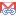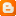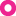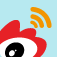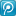### 3.4.15.Operator (LIKE)

With the experience on previous formula derivation, the process is smooth in the LIKE derivation. I directly deduce the formula with mixed data types and mixed NDV (In fact, conclusion in the article told us the selectivity of LIKE is same as the one of >, both is 1/20. It can be proved by following test):

 A:160 B:80 C:100 D:200 E:400 A:VARCHAR2 B:NUMBER C:DATE D:DATE E:NUMBER EFFQRYCOLNUM 0 FLTCOLNUM FILTER COST_CPU CPU_A TYPFAC CACULATED 1 a like :v1 257121440 7121440 250000000 250 2 a like :v1 and b like :v3 287121440 7121440 280000000 280 3 a like :v1 and b like :v2 and c like :v3 307996440 7121440 300875000 300.875 4 a like :v1 and b like :v2 and c like :v3 d like :v4 328040190 7121440 320918750 320.91875

From the test, the final rule is that the selectivity (1/NDV) is a fixed number, 1/20; however, it will increase the COLTYPFAC by 50. For example, the third one in above data set, the calculation is,

130+3*20+100*1+200*(1/20)+350*(1/20)^2 = 300.875

Then take the effective query columns into account,

 EFFQRYCOLNUM 1 FLTCOLNUM FILTER COST_CPU CPU_A TYPFAC CACULATED 1 a like :v1 258121440 7121440 251000000 251 2 a like :v1 and b like :v3 287171440 7121440 280050000 280.05 3 a like :v1 and b like :v2 and c like :v3 307998940 7121440 300877500 300.8775 4 a like :v1 and b like :v2 and c like :v3 d like :v4 328040310 7121440 320918870 320.91887 EFFQRYCOLNUM 2 FLTCOLNUM FILTER COST_CPU CPU_A TYPFAC CACULATED 1 a like :v1 287221440 7121440 280100000 280.1 2 a like :v1 and b like :v3 308001440 7121440 300880000 300.88 3 a like :v1 and b like :v2 and c like :v3 328040430 7121440 320918990 320.91899 4 a like :v1 and b like :v2 and c like :v3 d like :v4 259121440 7121440 252000000 252

Combine with the rule above, all of the results matched the results calculated by formula 18.

### 3.4.16.Other operators (<>, IN and NOT IN)

Base on the conclusion in , the selectivity of the other operators with bind variable is,

<>: 1/NDV

IN: (variable number)*(1/NDV)

NOT IN: (1-1/NDV)^(variable number)

Following the way I worked on < & =, it’s not hard to find the formulas.

#### 3.4.16.1.Operator (<>)

Here is the test data for <>:

 A:160 B:80 C:100 D:200 E:400 A:VARCHAR2 B:NUMBER C:DATE D:DATE E:NUMBER EFFQRYCOLNUM 0 FLTCOLNUM FILTER COST_CPU CPU_A CPU_B TYPFAC CACULATED 1 a <> :v1 207121440 7121440 200000000 200 200.00 EFFQRYCOLNUM 2 FLTCOLNUM FILTER COST_CPU CPU_A CPU_B TYPFAC CACULATED 1 a <> :v1 246871440 7121440 239750000 239.75 239.75 2 a <> :v1 and b <> :v3 415437060 7121440 408315620 408.31562 408.31562 3 a <> :v1 and b <> :v2 and c <> :v3 729442978 7121440 722321538 722.321538 722.3215375 4 a <> :v1 and b <> :v2 and c <> :v3 d <> :v4 1040703111 7121440 1033581671 1033.581671 1033.581671

As said, the selectivity of <> is (1-1/NDV). Base on this, the data above are matched the result calculated by formula 18. Take the third one as example,

130+3*20+50*1+150*(1-1/160)+300*(1-1/160)*(1-1/80)+2*20*MAX(1,ROUND((1-1/160)*(1-1/80)*(1-1/100)*1000000,0))/1000000 = 722.3215375

#### 3.4.16.2.Operator (IN)

After involved IN, it not only affected selectivity, but also affected the COLTYPFAC.

 A:160 B:80 C:100 D:200 E:400 A:VARCHAR2